# Recursion Chapter 11 1 Introduction to Recursion Sometimes

• Slides: 41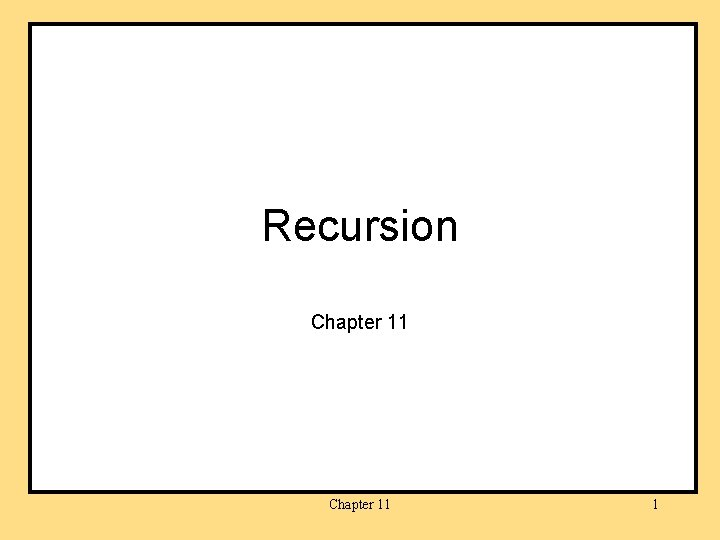Recursion Chapter 11 1Introduction to Recursion • Sometimes it is possible and useful to define a method in terms of itself. • A Java method definition is recursive if it contains an invocation of itself. • The method continues to call itself, with ever simpler cases, until a base case is reached which can be resolved without any subsequent recursive calls. Chapter 11 5Example: Search for a Name in a Phone Book • Open the phone book to the middle. • If the name is on this page, you’re done. • If the name alphabetically precedes the names on this page, use the same approach to search for the name in the first half of the phone book. • Otherwise, use the same approach to search for the name in the second half of the phone book. Chapter 11 6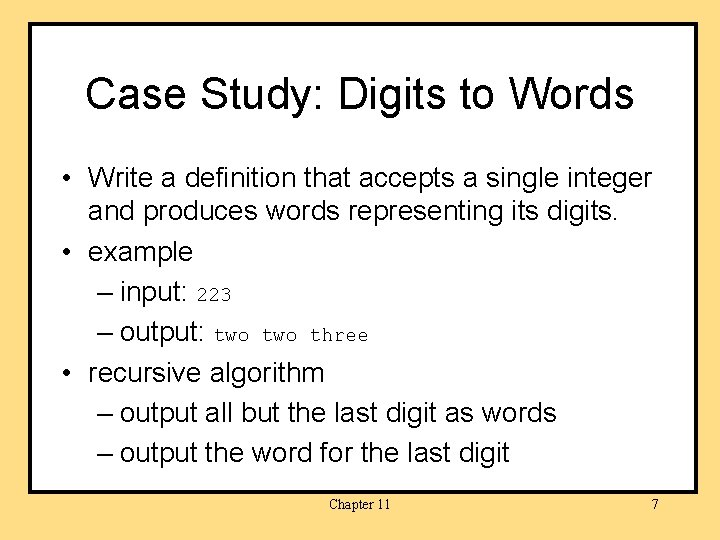Case Study: Digits to Words • Write a definition that accepts a single integer and produces words representing its digits. • example – input: 223 – output: two three • recursive algorithm – output all but the last digit as words – output the word for the last digit Chapter 11 7Case Study: Digits to Words, cont. • class Recursion. Demo Chapter 11 8Case Study: Digits to Words, cont. • class Recursion. Demo, contd. Chapter 11 9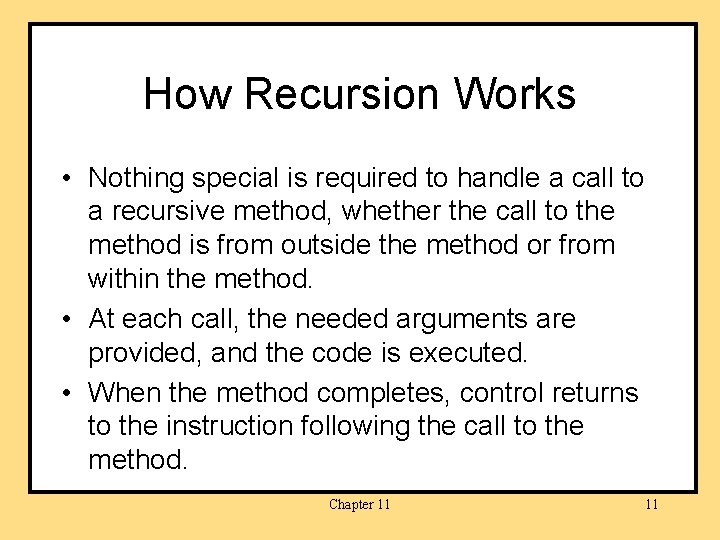How Recursion Works • Nothing special is required to handle a call to a recursive method, whether the call to the method is from outside the method or from within the method. • At each call, the needed arguments are provided, and the code is executed. • When the method completes, control returns to the instruction following the call to the method. Chapter 11 11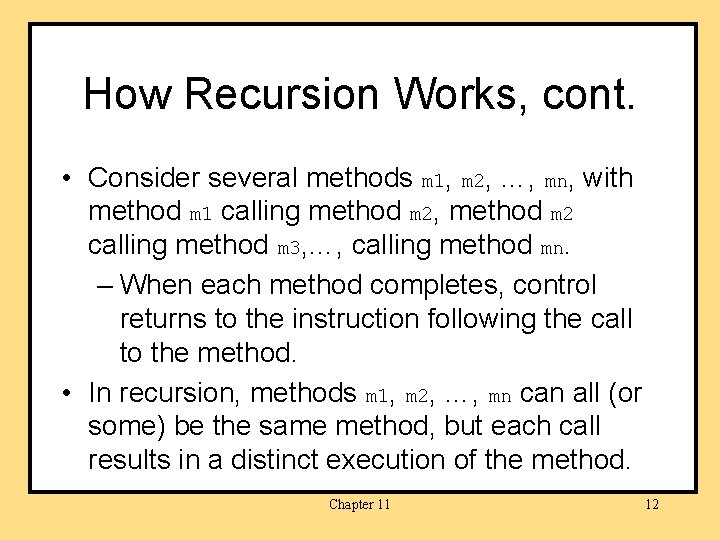How Recursion Works, cont. • Consider several methods m 1, m 2, …, mn, with method m 1 calling method m 2, method m 2 calling method m 3, …, calling method mn. – When each method completes, control returns to the instruction following the call to the method. • In recursion, methods m 1, m 2, …, mn can all (or some) be the same method, but each call results in a distinct execution of the method. Chapter 11 12How Recursion Works, cont. • As always, method m 1 cannot complete execution until method m 2 completes execution, method m 2 cannot complete execution until method m 3 completes execution, … until method mn completes execution. • If method mn represents a stopping case, it can complete execution, …, then method m 2 can complete execution, then method m 1 can complete execution. Chapter 11 13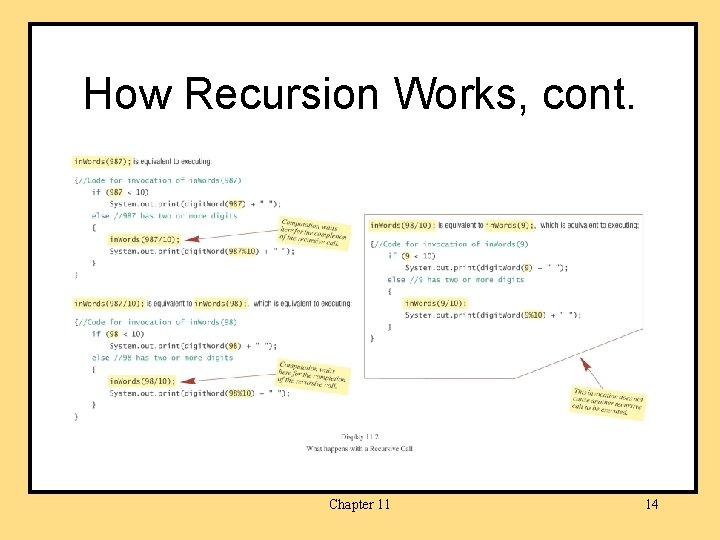How Recursion Works, cont. Chapter 11 14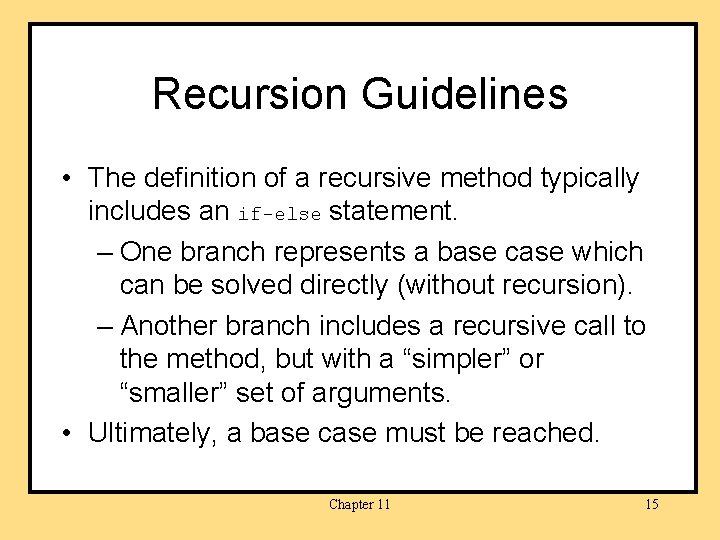Recursion Guidelines • The definition of a recursive method typically includes an if-else statement. – One branch represents a base case which can be solved directly (without recursion). – Another branch includes a recursive call to the method, but with a “simpler” or “smaller” set of arguments. • Ultimately, a base case must be reached. Chapter 11 15Infinite Recursion • If the recursive invocation inside the method does not use a “simpler” or “smaller” parameter, a base case may never be reached. • Such a method continues to call itself forever (or at least until the resources of the computer are exhausted as a consequence of stack overflow). • This is called infinite recursion. Chapter 11 16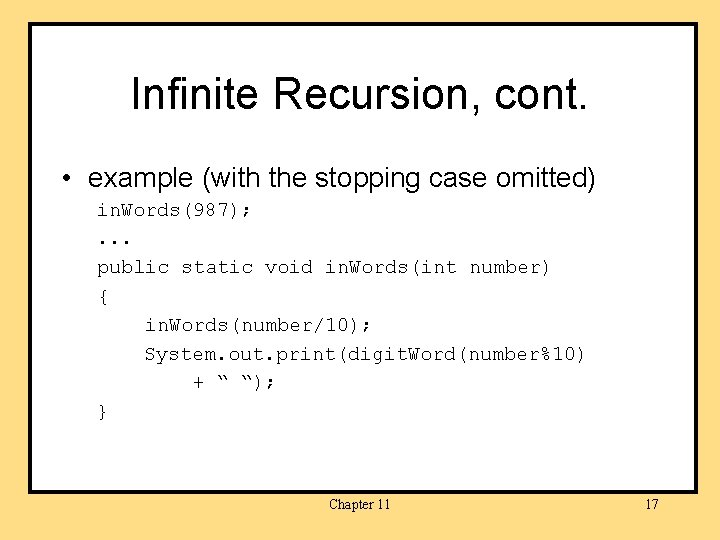Infinite Recursion, cont. • example (with the stopping case omitted) in. Words(987); . . . public static void in. Words(int number) { in. Words(number/10); System. out. print(digit. Word(number%10) + “ “); } Chapter 11 17Recursion vs. Iteration • Any recursive method can be rewritten without using recursion (but in some cases this may be very complicated). • Typically, a loop is used in place of the recursion. • The resulting method is referred to as the iterative version. Chapter 11 18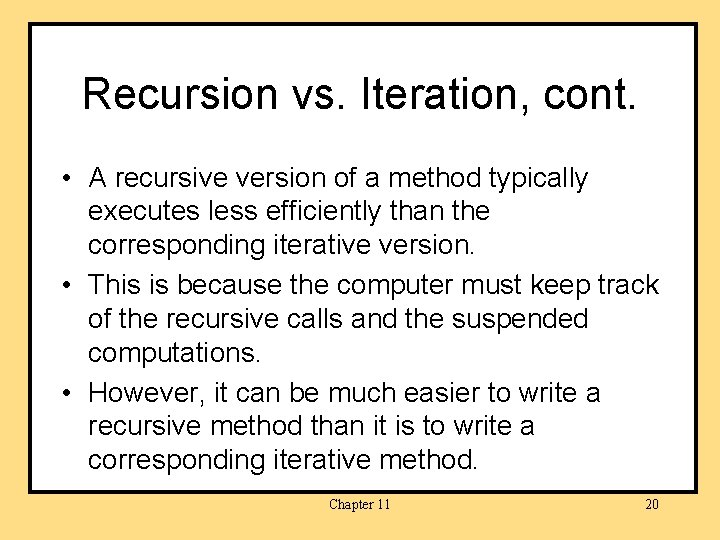Recursion vs. Iteration, cont. • A recursive version of a method typically executes less efficiently than the corresponding iterative version. • This is because the computer must keep track of the recursive calls and the suspended computations. • However, it can be much easier to write a recursive method than it is to write a corresponding iterative method. Chapter 11 20Recursive Methods That Return a Value • A recursive method can be a void method or it can return a value. • At least one branch inside the recursive method can compute and return a value by making a chain of recursive calls. • Consider, for example, a method that takes a single int argument and returns the number of zeros in the argument. Chapter 11 21Recursive Methods That Return a Value, cont. • If n is two or more digits long, then the number of zero digits in n is (the number of zeros in n with the last digit removed) plus an additional one if the last digit is a zero. Chapter 11 22Recursive Methods That Return a Value, cont. • class Recursion. Demo 2 Chapter 11 23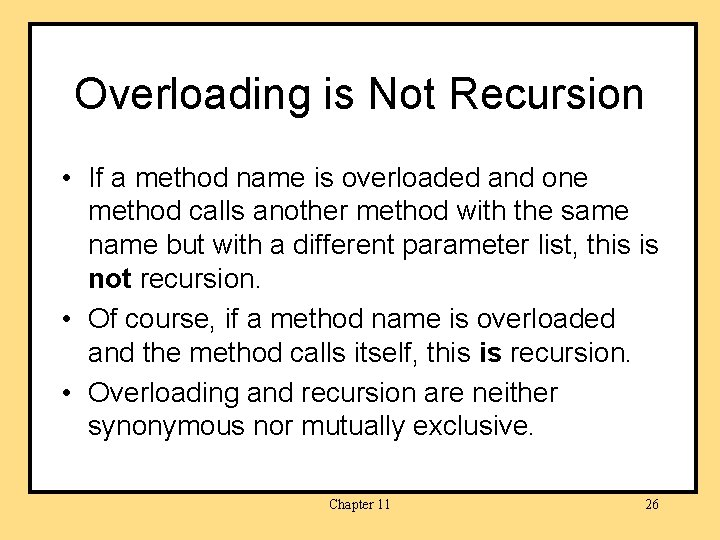Overloading is Not Recursion • If a method name is overloaded and one method calls another method with the same name but with a different parameter list, this is not recursion. • Of course, if a method name is overloaded and the method calls itself, this is recursion. • Overloading and recursion are neither synonymous nor mutually exclusive. Chapter 11 26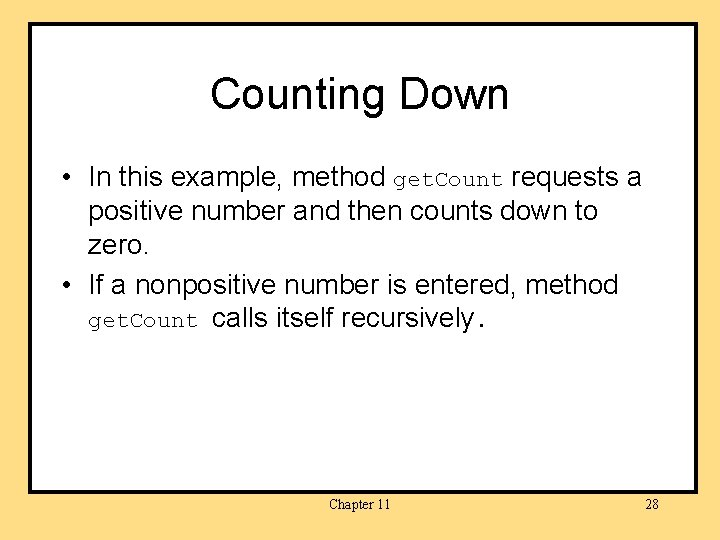Counting Down • In this example, method get. Count requests a positive number and then counts down to zero. • If a nonpositive number is entered, method get. Count calls itself recursively. Chapter 11 28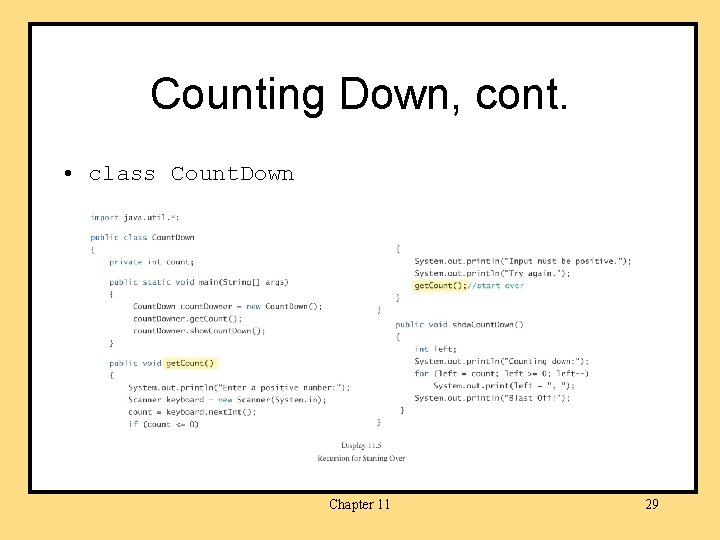Counting Down, cont. • class Count. Down Chapter 11 29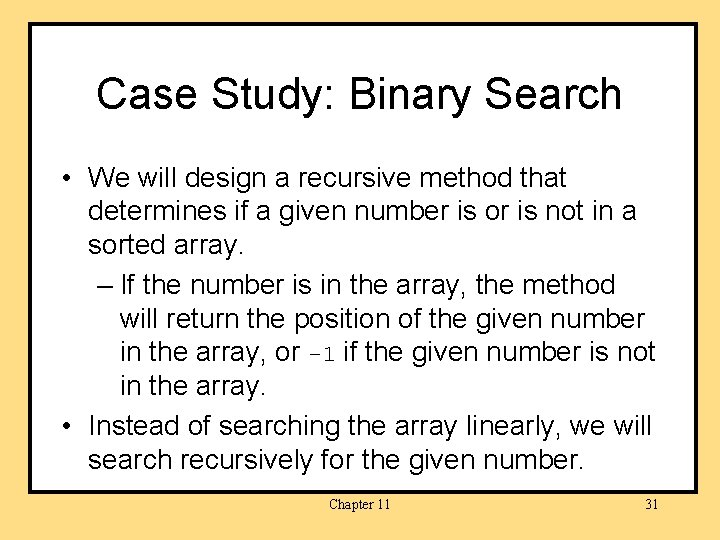Case Study: Binary Search • We will design a recursive method that determines if a given number is or is not in a sorted array. – If the number is in the array, the method will return the position of the given number in the array, or -1 if the given number is not in the array. • Instead of searching the array linearly, we will search recursively for the given number. Chapter 11 31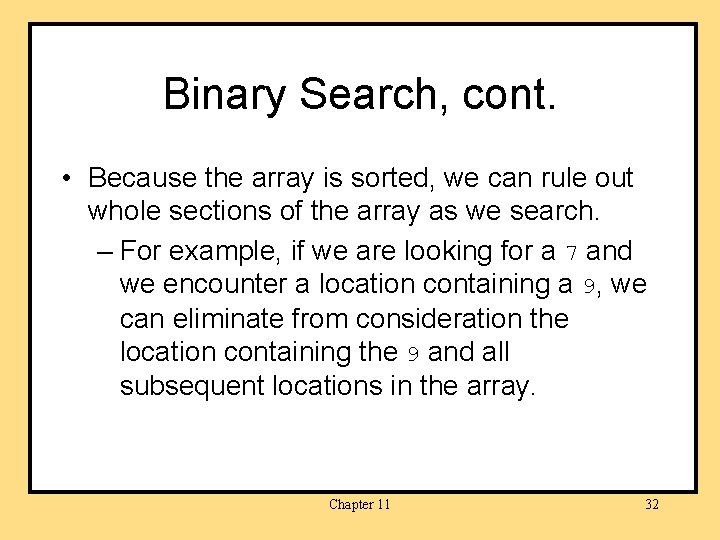Binary Search, cont. • Because the array is sorted, we can rule out whole sections of the array as we search. – For example, if we are looking for a 7 and we encounter a location containing a 9, we can eliminate from consideration the location containing the 9 and all subsequent locations in the array. Chapter 11 32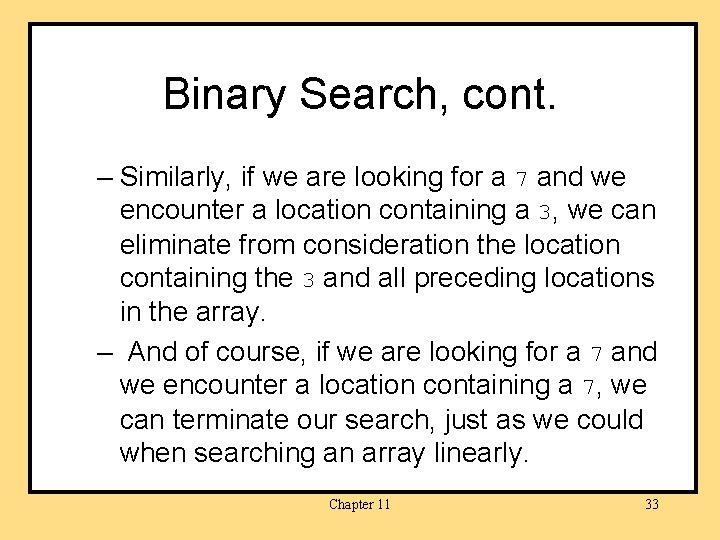Binary Search, cont. – Similarly, if we are looking for a 7 and we encounter a location containing a 3, we can eliminate from consideration the location containing the 3 and all preceding locations in the array. – And of course, if we are looking for a 7 and we encounter a location containing a 7, we can terminate our search, just as we could when searching an array linearly. Chapter 11 33Binary Search, cont. • We can begin our search by examining an element mid in the middle of the array. • pseudocode, first draft: mid = (0 + a. length-1)/2 if (target == a[mid]) return mid; else if (target < a[mid] search a through a[mid-1] else search a[mid + 1] through a[a. length - 1] Chapter 11 34Binary Search, cont. • pseudocode, generalized for recursive calls: mid = (first + last)/2 if (target == a[mid]) return mid; else if (target < a[mid] search a[first] through a[mid-1] else search a[mid + 1] through a[last] Chapter 11 35Binary Search, cont. • But what if the number is not in the array? – first eventually becomes larger than last and we can terminate the search. • Our pseudocode needs to be amended to test if first has become larger than last. Chapter 11 36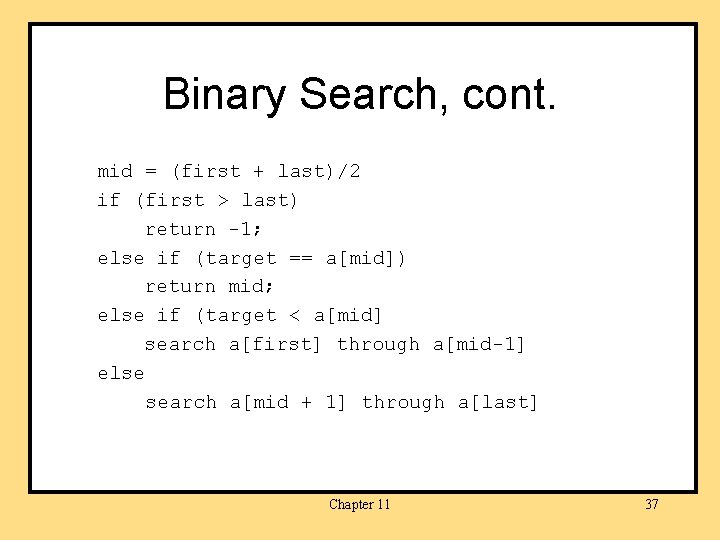Binary Search, cont. mid = (first + last)/2 if (first > last) return -1; else if (target == a[mid]) return mid; else if (target < a[mid] search a[first] through a[mid-1] else search a[mid + 1] through a[last] Chapter 11 37Binary Search, cont. • class Array. Searcher Chapter 11 38Binary Search, cont. Chapter 11 39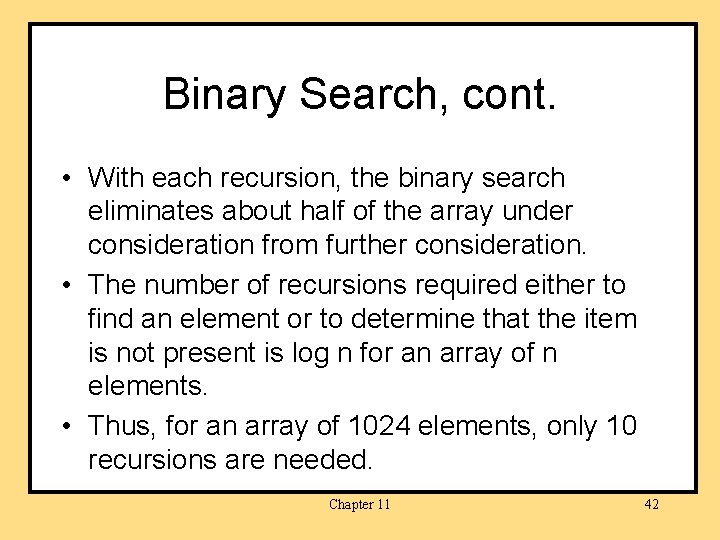Binary Search, cont. • With each recursion, the binary search eliminates about half of the array under consideration from further consideration. • The number of recursions required either to find an element or to determine that the item is not present is log n for an array of n elements. • Thus, for an array of 1024 elements, only 10 recursions are needed. Chapter 11 42Merge Sort • Efficient sorting algorithms often are stated recursively. • One such sort, merge sort, can be used to sort an array of items. • Merge sort takes a “divide and conquer” approach. – The array is divided in halves and the halves are sorted recursively. – Sorted subarrays are merged to form a larger sorted array. Chapter 11 43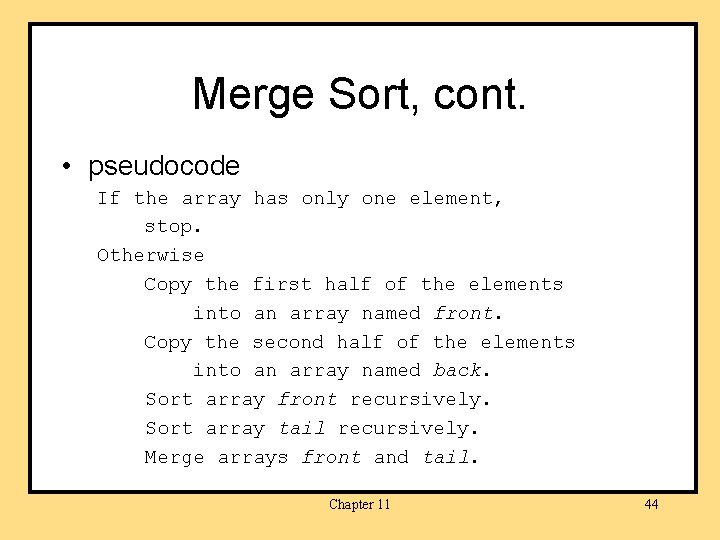Merge Sort, cont. • pseudocode If the array has only one element, stop. Otherwise Copy the first half of the elements into an array named front. Copy the second half of the elements into an array named back. Sort array front recursively. Sort array tail recursively. Merge arrays front and tail. Chapter 11 44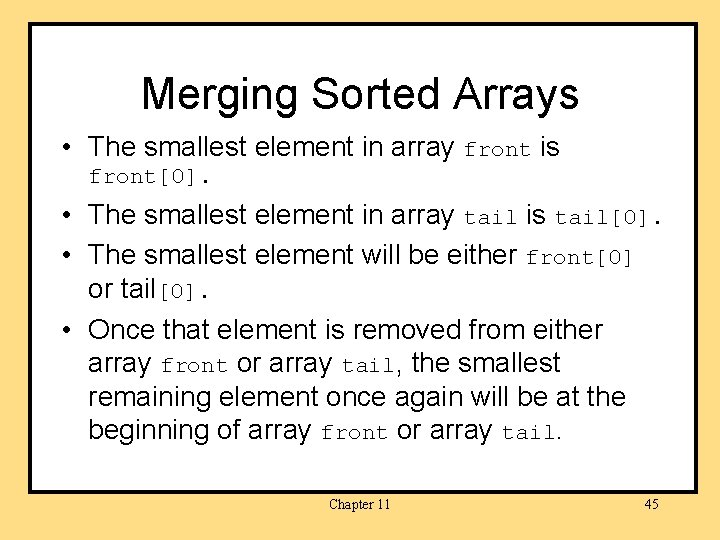Merging Sorted Arrays • The smallest element in array front is front. • The smallest element in array tail is tail. • The smallest element will be either front or tail. • Once that element is removed from either array front or array tail, the smallest remaining element once again will be at the beginning of array front or array tail. Chapter 11 45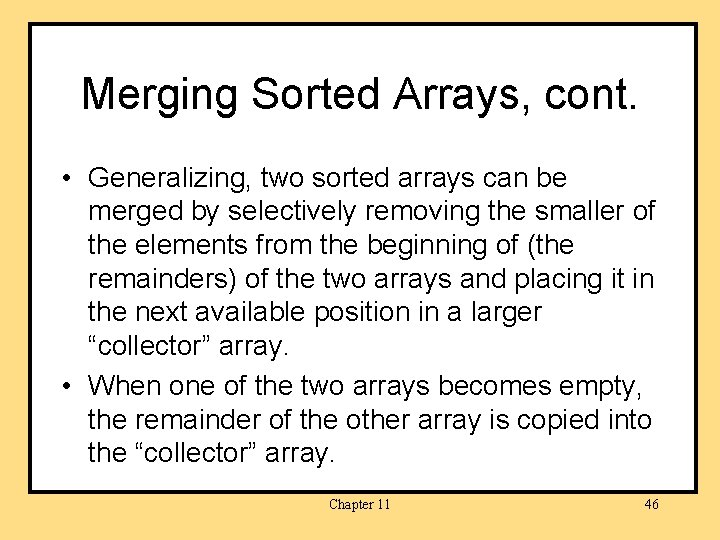Merging Sorted Arrays, cont. • Generalizing, two sorted arrays can be merged by selectively removing the smaller of the elements from the beginning of (the remainders) of the two arrays and placing it in the next available position in a larger “collector” array. • When one of the two arrays becomes empty, the remainder of the other array is copied into the “collector” array. Chapter 11 46Merging Sorted Arrays, cont. int front. Index = 0, tail. Index = 0, a. Index = 0; while ((front. Index < front. length) && (tail. Index < tail. length)) { if(front[front. Index] < tail[tail. Index]} { a[a. Index] = front[front. Index]; a. Index++; front. Index++; } Chapter 11 47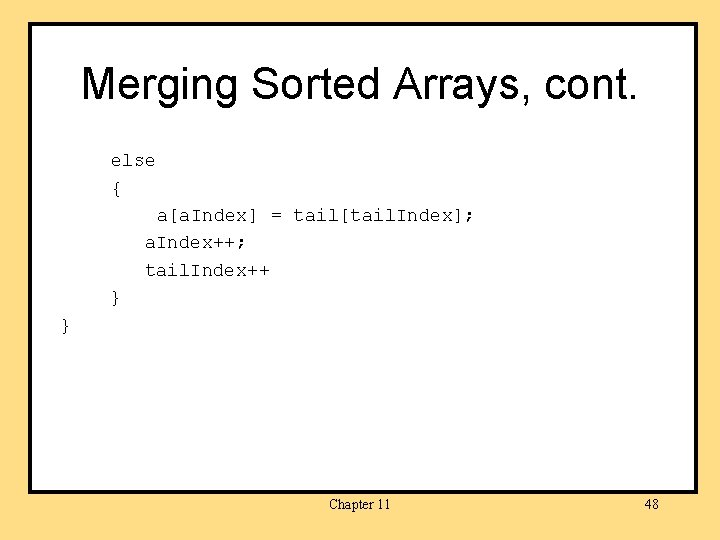Merging Sorted Arrays, cont. else { a[a. Index] = tail[tail. Index]; a. Index++; tail. Index++ } } Chapter 11 48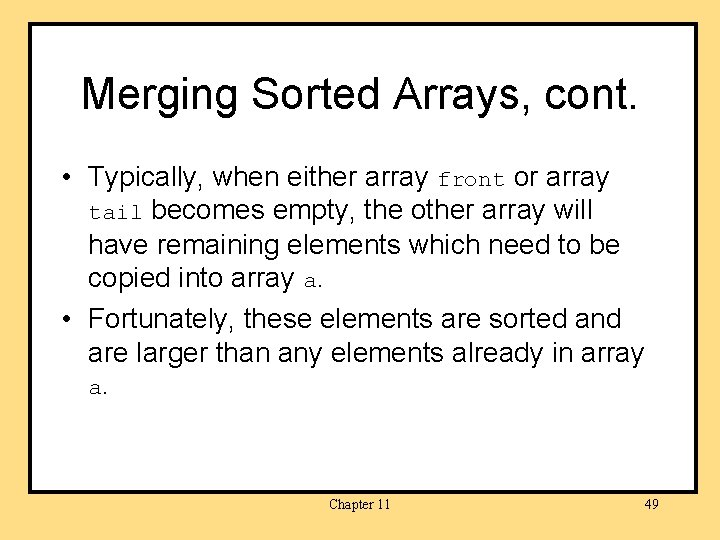Merging Sorted Arrays, cont. • Typically, when either array front or array tail becomes empty, the other array will have remaining elements which need to be copied into array a. • Fortunately, these elements are sorted and are larger than any elements already in array a. Chapter 11 49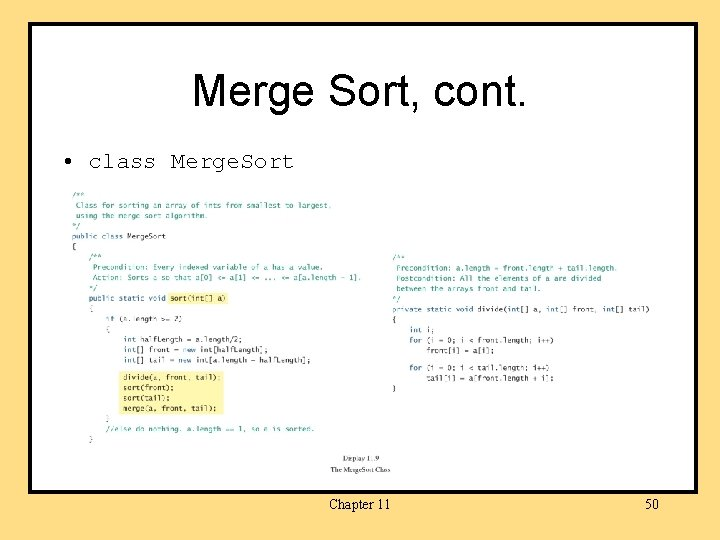Merge Sort, cont. • class Merge. Sort Chapter 11 50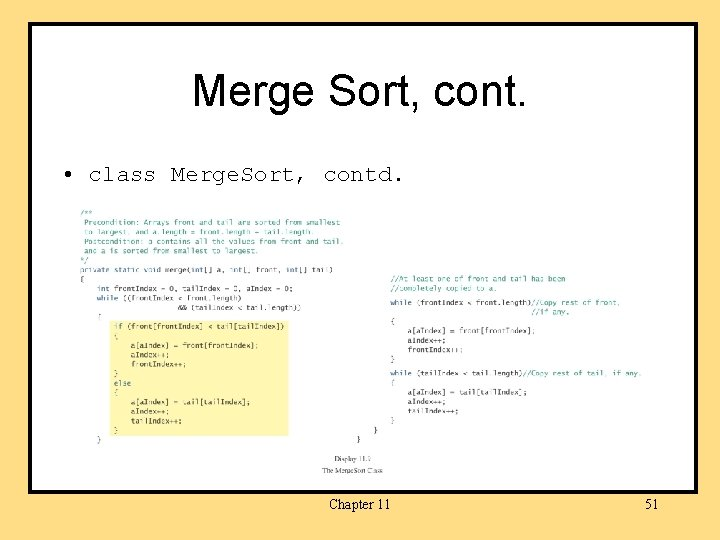Merge Sort, cont. • class Merge. Sort, contd. Chapter 11 51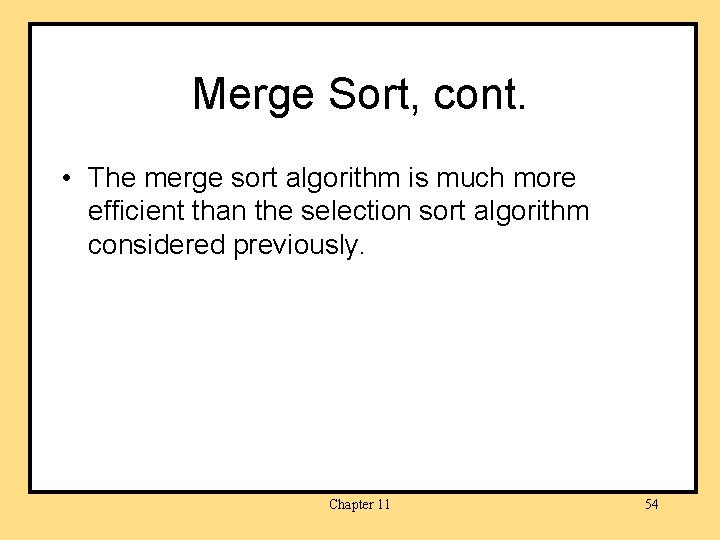Merge Sort, cont. • The merge sort algorithm is much more efficient than the selection sort algorithm considered previously. Chapter 11 54